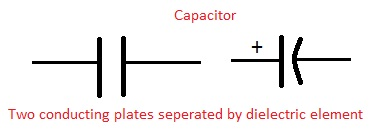# Arduino Capacitance meterBy using arduino digital pins and serial monitor we can measure unknown capacitor value.

This is calculation based technique, by charging and discharging capacitor through known value resistor and by calculating the time delay we can measure unknown capacitance value in farad.

C = Tc/R

Tc– time constant of capacitor (in seconds)

R – resistance of the circuit (in ohms)

C – the capacitance

The same method is used in arduino circuit to find out unknown capacitor value. Here digital pin 13 used as charge pin for capacitor and digital pin 11 acts as discharge pin the time constant output fed into analog read pin A0.

## Arduino Code

```// Initialize Pins
int analogPin = 0;
int chargePin = 13;
int dischargePin = 11; //speeds up discharging process, not necessary though

// Initialize Resistor
int resistorValue = 560;

// Initialize Timer
unsigned long startTime;
unsigned long elapsedTime;

// Initialize Capacitance Variables

void setup()
{
pinMode(chargePin, OUTPUT);
digitalWrite(chargePin, LOW);
Serial.begin(9600); // Necessary to print data to serial monitor over USB
}

void loop()
{
digitalWrite(chargePin, HIGH); // Begins charging the capacitor
startTime = millis(); // Begins the timer

{
// Does nothing until capacitor reaches 63.2% of total voltage
}

elapsedTime= millis() - startTime; // Determines how much time it took to charge capacitor
microFarads = ((float)elapsedTime / resistorValue) * 1000;
Serial.print(elapsedTime);
Serial.print(" mS    ");

if (microFarads > 1) // Determines if units should be micro or nano and prints accordingly
{
}

else
{
delay(500);
}

digitalWrite(chargePin, LOW); // Stops charging capacitor
pinMode(dischargePin, OUTPUT);
digitalWrite(dischargePin, LOW); // Allows capacitor to discharge
{
// Do nothing until capacitor is discharged
}

pinMode(dischargePin, INPUT); // Prevents capacitor from discharging
}

```